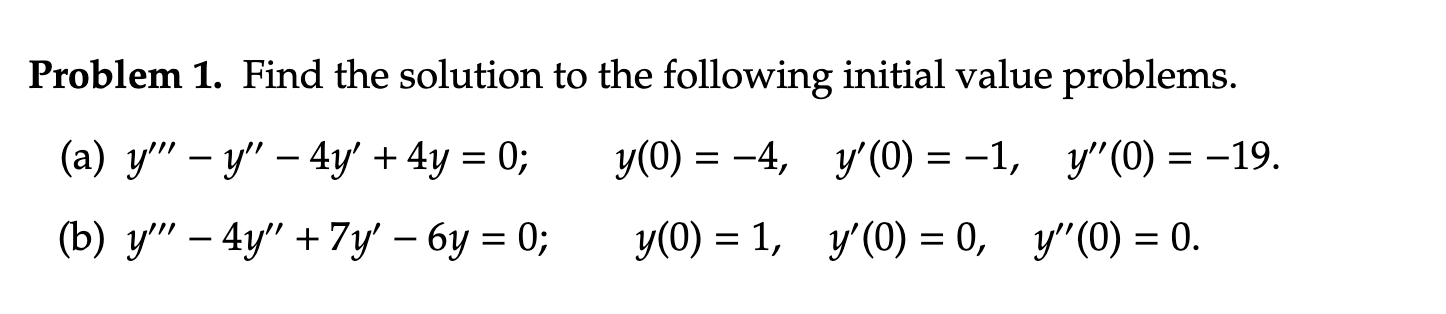# Problem 1. Find the solution to the following initial value problems. (a) y'" – y" –...

###### Question:Problem 1. Find the solution to the following initial value problems. (a) y'" – y" – 4y' + 4y = 0; y(0) = -4, y'(0) = -1, y"(0) = -19. (b) y'' – 4y"' + 7y – by = 0; y(0) = 1, y'(0) = 0, y"(O) = 0.

#### Similar Solved Questions

##### The lever below is constructed of AISI 1050 cold-drawn steel. It is assumed that bar "DC"...
The lever below is constructed of AISI 1050 cold-drawn steel. It is assumed that bar "DC" is strong enough and hence not part of the problem. The force "F" fluctuates between 50 and 250 lbf. Calculate the factor of safety against yielding and fatigue for a desired reliability of 99.9...
##### (2x)/(x^(2)+1)^(2)>0?
(2x)/(x^(2)+1)^(2)>0?...
##### 1. 0 points) Signai flow below represents the processin a common mechatronics system 0011 0010 0100...
1. 0 points) Signai flow below represents the processin a common mechatronics system 0011 0010 0100 0011 0010 1011 0011 0011 1100 0011 0101 0111 A/D computer/ converter memory sample sensor buffer aimpliticer low-pass filer&hold (a) The function of the buffer amplifier is to (b) Although the flo...